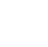# Java Constants

Constants in Java refer to fixed values that do not change during the execution of the program. A constant is a variable whose value cannot change once it has been assigned.

To define a variable as a constant, we just need to add the keyword “final” in front of the variable declaration.

Syntax:

``` final data_type = value; ```

Example:

``` final float pi = 3.14f; ```

The above statement declares the float variable “pi” as a constant with a value of 3.14f. We cannot change the value of "pi" at any point in time in the program.

## Types of Constants

i) Integer Constants : It refers to sequence of digits . There are three types of integers namely decimal integer , octal integer and hexadecimal integer.

``` final int num1 =  225; // decimal integer begins with non zero digitfinal int num2 =  035; // octal integer begins with 0final int num3 =  0x2; // hexadecimal integer begins with "0x" or "0X" ```

ii) Real Constants: Integer numbers are inadequate to represent quantities that are very continuously, such as temperatures, prices, heights ,etc. These numbers are represented by fractional parts. Such numbers are called real constants.

``` final int num1 = 435.36;// real constants are represented by fractional parts. ```

iii) Single Character Constants : It contains a single character enclosed within a pair of single quote marks.

``` final char alphabet1 = 'A'; // 'A' is a single character constant enclosed with single quote. final char num1 = '5'; // '5' is single character constant enclosed with single quote. ```

iv) String Constants: It contains a single character enclosed within a pair of double quote marks. Example: “hello", “2000”, “7+5”.

``` final String greeting = "Hello"; // "Hello" is a string constant enclosed with double quotes final String num = "20"; // "20" is a string constant enclosed with double quotes ```